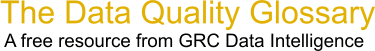Choose Index below for a list of all words and phrases defined in this glossary.

# Pearson's Correlation

index | Index

## Pearson's Correlation - definition(s)

Pearson's Correlation - Pearson's correlation reflects the degree of linear relationship between two variables.

Pearson's correlation coefficient (r) for continuous (interval level) data ranges from -1 to +1:

Positive correlation indicates that both variables increase or decrease together, whereas negative correlation indicates that as one variable increases, so the other decreases, and vice versa.

[Category=Data Quality ]

Source: iSixSigma, 10 February 2011 09:58:47, http://www.isixsigma.com/index.php?option=com_glossaryData Quality Glossary.  A free resource from GRC Data Intelligence. For comments, questions or feedback: dqglossary@grcdi.nl